# Common Core: 7th Grade Math : Subtract Rational Numbers and Understand The Absolute Value of Their Difference: CCSS.Math.Content.7.NS.A.1c

## Example Questions

← Previous 1 3

### Example Question #1 : Negative Numbers

Solve: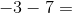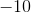Explanation: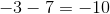The problem indicates that the result isunits more negative than, which is.

### Example Question #1 : Subtract Rational Numbers And Understand The Absolute Value Of Their Difference: Ccss.Math.Content.7.Ns.A.1c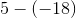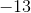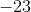Explanation:

When we subtract a negative number, the sign turns positive. It becomes an addition problem.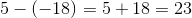.

### Example Question #1 : Subtract Rational Numbers And Understand The Absolute Value Of Their Difference: Ccss.Math.Content.7.Ns.A.1c

Solve: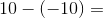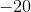Explanation:

Remember that subtracting a negative number is just like adding the positive of that number, because you move to the right (more positive) on the number line. Therefore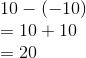### Example Question #1 : Subtract Rational Numbers And Understand The Absolute Value Of Their Difference: Ccss.Math.Content.7.Ns.A.1c

Subtract: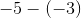Explanation:

Distribute the negative sign through the parentheses.  Double negatives equal a positive.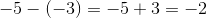The answer is.

### Example Question #5 : Subtract Rational Numbers And Understand The Absolute Value Of Their Difference: Ccss.Math.Content.7.Ns.A.1c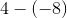Explanation:

When minus signs meet, the sign becomes positive. This becomes an addition problem. Answer is.

### Example Question #1 : Subtract Rational Numbers And Understand The Absolute Value Of Their Difference: Ccss.Math.Content.7.Ns.A.1c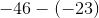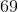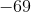Explanation:

When two minus signs meet, the sign becomes positive. Sinceis greater thanand is negative, our answer is negative. We treat as a subtraction problem. Answer is.

### Example Question #1 : Subtract Rational Numbers And Understand The Absolute Value Of Their Difference: Ccss.Math.Content.7.Ns.A.1c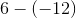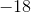Explanation:

Two minus signs become a plus sign. It's now an addition problem.

Add the ones digits together: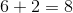Add the tens digits together: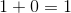Combine the ones and tens digit to get final answer.

Answer is.

### Example Question #1 : Subtract Rational Numbers And Understand The Absolute Value Of Their Difference: Ccss.Math.Content.7.Ns.A.1c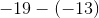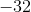Explanation:

Two minus signs become a plus sign. It's now an addition problem. Sinceis greater thanand is negative, our answer is negative.

We treat as a normal subtraction.

Answer is### Example Question #1 : Subtract Rational Numbers And Understand The Absolute Value Of Their Difference: Ccss.Math.Content.7.Ns.A.1c

Solve: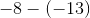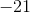Explanation:

Two minus signs becomes a plus sign. Sinceis greater thanand is positive, our answer is positive. We treat as a normal subtraction. Answer is.

### Example Question #71 : The Number System

Solve: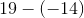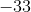Two minus signs become a plus sign. This is just simple addition. Answer is.